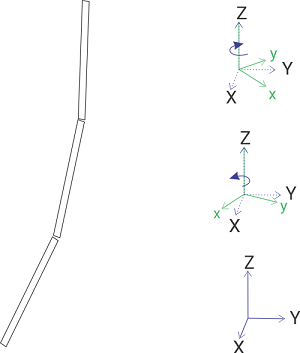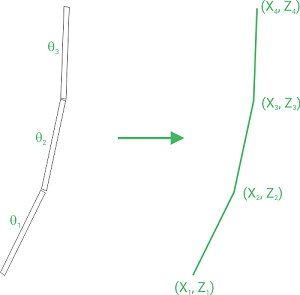Measurand's ShapeArray uses MEMS accelerometers to determine the tilt of individual segments with respect to gravity, as shown in the figure below. Using the tilt and the known segment length, it is possible to represent the tilted segment as a line segment in 3D space. Figure 1 shows a simplified example with only X and Z positions shown.Figure 1. Representing tilt in a segment as a set of line coordinates

After a ShapeArray is read, the raw data counts are stored in data files. For ShapeArrays connected to a data logger, the raw data files are organized into three columns per segment. These are the raw data counts for the tilt measurements of the board x, y, and z sensors. ShapeArray conversion protocols use the measured tilts and various calibration factors to determine the shape of the instrument for a particular reading.

The board in each segment have a random alignment which is aligned in software using the roll calibration information created during the manufacturing process. During the conversion process, the MEMS accelerometer axes are aligned in software according to calibration files created during manufacturing, as shown in the figure below. This forms a set of data with a consistent orthogonal set for X, Y, and Z. The X data is presented so that it aligns with the X-mark provided on the ShapeArray. For ShapeArrays with magnetometers, the X data is instead aligned with magnetic north.Figure 2. Aligning sensor x and y to the instrument X and Y axes for the ShapeArray

Data for a ShapeArray are represented as either a 2D polyline for horizontal or convergence applications or, more commonly, as a 3D polyline for vertical applications. When data is exported, the tilts measured for the polyline are converted into Cartesian coordinates as shown in the figure below.Figure 3. ShapeArray measured tilts in the X plane are shown on the left. The export values for coordinates in the X  plane are shown on the right.

X, Y, and Z coordinates at the segment ends of the instrument are exported as a comma separated values data file. In the exported data file, the (0, 0, 0) coordinate corresponds to the reference end of the instrument. The next set of coordinates is for the adjacent joint. This arrangement continues until the other end of the instrument is reached. For a ShapeArray installed in a vertical borehole, with the reference at the bottom, the (0, 0, 0) coordinate corresponds the bottom of the borehole. In order to determine the displacement of the ShapeArray over time, the first reading (baseline) is subtracted from the following readings.

Displacement and deviation measurements are discussed in more detail in Understanding Deviation and Displacement in ShapeArray Data.